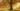# 掌握 JavaScript 函数参数

## 函数参数

JavaScript 函数可以有任意数量的参数。让我们分别声明参数个数为 0，1 和 2 个的函数：

``````// 0 个参数
function zero() {
return 0;
}

// 1 个参数
function identity(param) {
return param;
}

// 2 个参数
function sum(param1, param2) {
return param1 + param2;
}

zero(); // => 0
identity(1); // => 1
sum(1, 2); // => 3``````

``````function sum(param1, param2) {
console.log(param1); // 1
console.log(param2); // undefined
return param1 + param2;
}

sum(1); // => NaN``````

`param1 + param2` 等价于 `1 + undefined`，所以结果为 `NaN`

``````function sum(param1, param2) {
if (param2 === undefined) {
param2 = 0;
}
return param1 + param2;
}

sum(1); // => 1``````

## 默认参数

ES2015 默认参数功能允许使用默认值初始化参数。这是比上面例子更好且更简洁的方式。

``````function sum(param1, param2 = 0) {
console.log(param2); // => 0
return param1 + param2;
}

sum(1); // => 1
sum(1, undefined); // => 1``````

## 参数解构

``````function greet({ name }) {
return `Hello, \${name}!`;
}

const person = { name: 'John Smith' };
greet(person); // => 'Hello, John Smith!'``````

`{ name }` 是一个从对象解构出的参数。可以很容易组合使用默认参数和解构：

``````function greetWithDefault({ name = 'Unknown' } = {}) {
return `Hello, \${name}!`;
}

greetWithDefault(); // => 'Hello, Unknown!'``````

`{ name = 'Unknown' } = {}` 默认为一个空对象。

``````function greeFirstPerson([{ name }]) {
return `Hello, \${name}!`;
}

const persons = [{ name: 'John Smith' }, { name: 'Jane Doe' }];
greeFirstPerson(persons); // => 'Hello, John Smith!'``````

`[{ name }]` 参数解构更加复杂。它首先提取数组的第一项，然后读取该项的 `name` 属性。

## 参数对象

JavaScript 函数另一个好功能是使用可变数量参数执行同一函数。

``````function sumArgs() {
console.log(arguments);
// -> { 0: 5, 1: 6, length: 2 }
let sum = 0;
for (let i = 0; i < arguments.length; i++) {
sum += arguments[i];
}
return sum;
}

sumArgs(5, 6); // => 11``````

`arguments` 包含函数执行时的参数。

`arguments` 是一个类数组对象(array-like object)，所以它不能使用所有的数组方法。

``````function outerFunction() {
const outerArguments = arguments;
return function innerFunction() {
// outFunction arguments
outerArguments;
};
}``````

### 箭头函数

``````const sumArgs = () => {
console.log(arguments);
return 0;
};

// throws: "Uncaught ReferenceError: arguments is not defined"
sumArgs();``````

`arguments` 没有声明在箭头函数内。但这不是大问题。我们可以使用更高效的扩展参数来访问箭头函数的所有参数。

## 扩展参数（Rest parameters）

ES2015 扩展参数让你收集函数的所有参数为数组。让我们使用扩展参数对参数求和：

``````function sumArgs(...numbers) {
console.log(numbers); // [5, 6]
return numbers.reduce((sum, number) => sum + number);
}

sumArgs(5, 6); // => 11``````

``````const sumArgs = (...numbers) => {
console.log(numbers); // [5, 6]
return numbers.reduce((sum, number) => sum + number);
};

sumArgs(5, 6); // => 11``````

``````function multiplyAndSumArgs(multiplier, ...numbers) {
console.log(multiplier); // 2
console.log(numbers); // [5, 6]
const sumArgs = numbers.reduce((sum, number) => sum + number);
return multiplier * sumArgs;
}

multiplyAndSumArgs(2, 5, 6); // => 22``````

`multiplier` 是参数值的第一个常规参数，然后 `...numbers` 包含所有剩余参数。

## 总结

JavaScript 解构的所有功能都可以应用于参数。您甚至可以将解构与默认参数结合使用。

`arguments` 是一个类数组的特殊对象，其中包含调用该函数所用的所有参数。